# Ratio in Simplest Form

In order to convert the given Ratio in Simplest Form, we should follow the following steps : –

• Find the HCF of both the numerator and denominator
• Dividing Both numbers by their HCF

Question 1:

Convert the ratio 60 : 35 in its simplest form.

Explanation

HCF of 60 and 35 is 5

Since, 60 : 35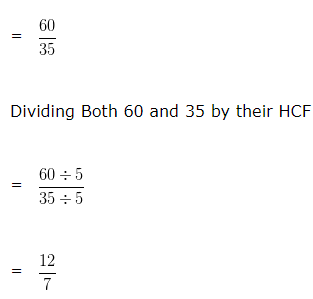= 12 : 7

Hence, the simplest form of 60 : 35 is 12 : 7

Question 2

Convert the ratio 18 : 36 in its simplest form.

Explanation

HCF of 18 and 36 is 18

Since, 18 : 36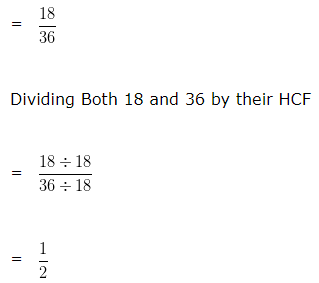= 1 : 2

Hence, the simplest form of 18 : 36 is 1 : 2

Question 3

Convert the ratio 25 : 35 in its simplest form.

Explanation

HCF of 25 and 35 is 5

Since, 25 : 35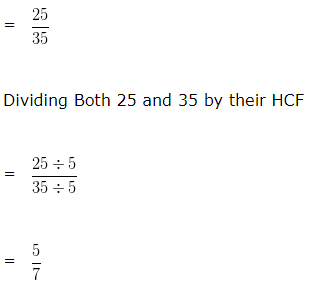= 5 : 7

Hence, the simplest form of 25 : 35 is 5 : 7

Question 4

Convert the ratio 78 : 12 in its simplest form.

Explanation

HCF of 78 and 12 is 6

Since, 78 : 12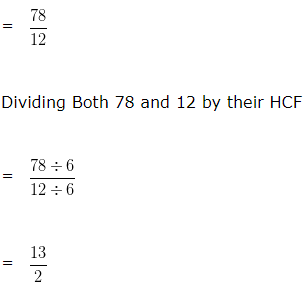= 13 : 2

Hence, the simplest form of 78 : 12 is 13 : 2

Question 5

Convert the ratio 25 : 75 in its simplest form.

Explanation

HCF of 25 and 75 is 25

Since, 25 : 75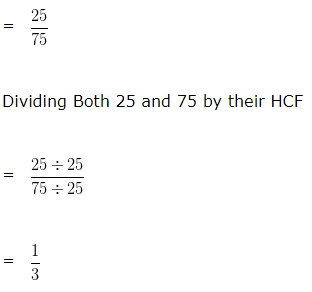= 1 : 3

Hence, the simplest form of 25 : 75 is 1 : 3